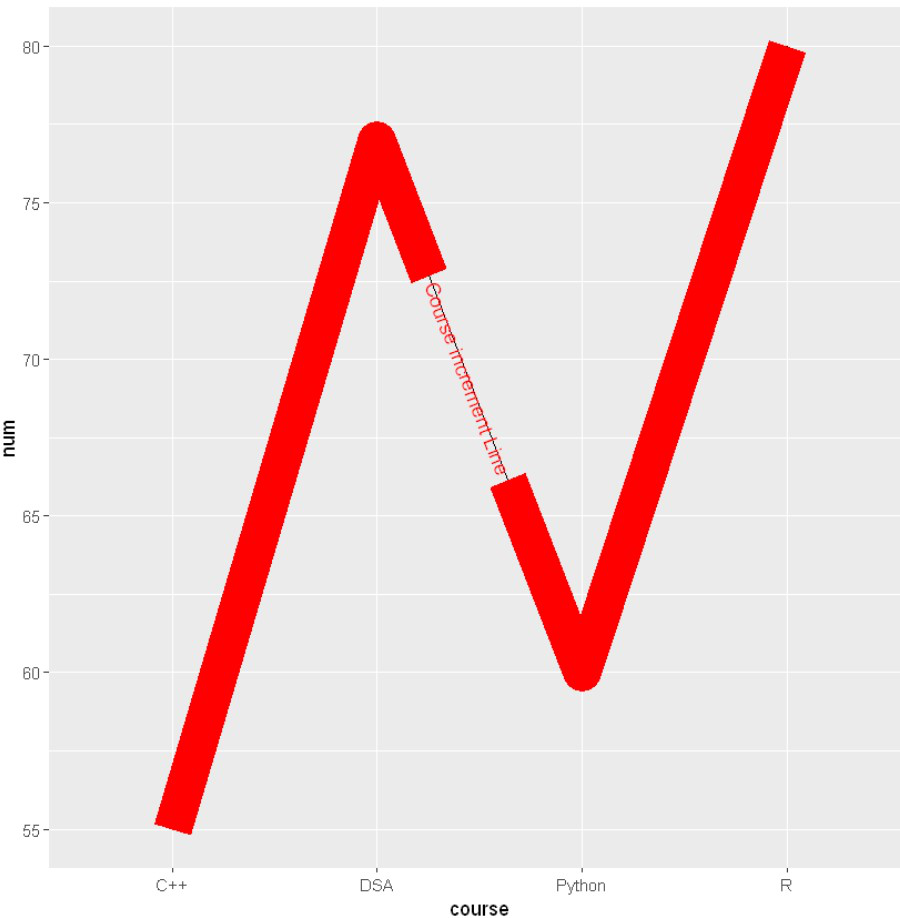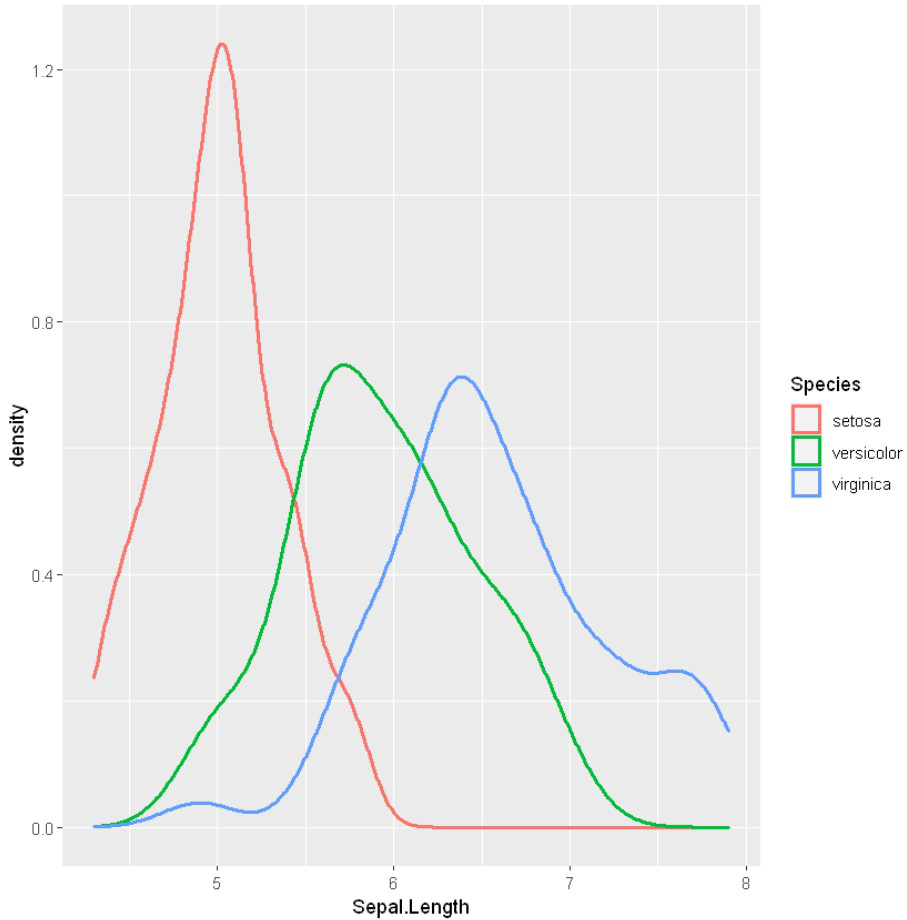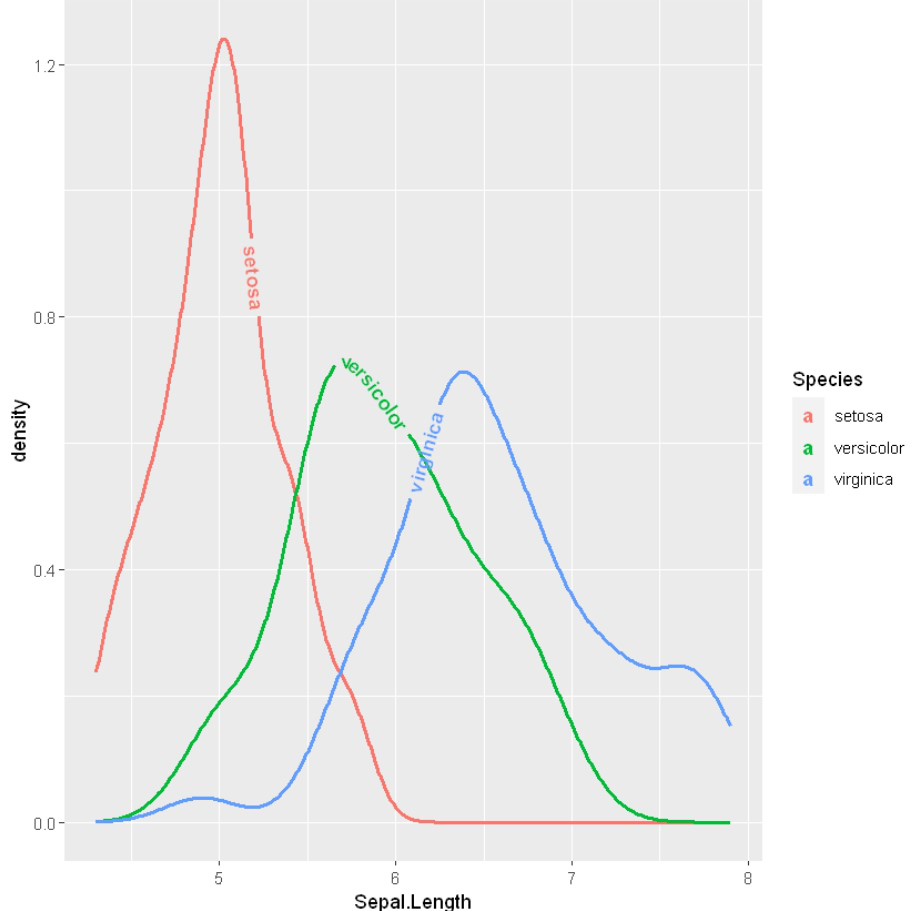GeeksforGeeks App
Open AppBrowser
Continue

# Labeling line plots with geomtextpath package in R

In this article, we are going to see how to use direct Labeling on line plots with geomtextpath Package in R Programming Language.

Geomtextpath is used to customize the labeling and using geomtextpath graph text follows any path, and it will remain correctly spaced and angled, even if you change the size and aspect ratio of your plotting device.

## Installation

To install this package type the below command in the terminal.

```install.packages("remotes")
remotes::install_github("AllanCameron/geomtextpath")```

## Method 1: With geom_textline() methods

Here, we are creating a dataframe and plotting a line plot using ggplot2 and then using geom_textline() methods from geomtextpath.

## R

 `library``(ggplot2)``library``(geomtextpath)`` ` `# Create data for chart``val <-``data.frame``(course=``c``(``'DSA'``,``'C++'``,``'R'``,``'Python'``),``                ``num=``c``(77,55,80,60))`` ` `# Basic Line``ggplot``(data=val, ``aes``(x=course, y=num, group=1)) +``geom_line``()+``geom_textline``(label = ``"Course increment Line"``, ``                ``color = ``"Red"``,``                ``linewidth=10) ``geom_point``()`## Method 2: With geom_density() methods

geom_density() is also used to add new labeling, first, we will create density lines and then apply geom_density.

## R

 `library``(``"ggplot2"``)`` ` `ggplot``(iris, ``aes``(Sepal.Length, colour = Species)) +``geom_density``(size=1)`

Output:## Method 3: With geomtextdensity() methods

Here we use the iris dataset to create density line and then we will use geomtextdensity() methods from geomtextpath

## R

 `library``(``"ggplot2"``)``library``(geomtextpath)`` ` `ggplot``(iris, ``aes``(Sepal.Length, colour = Species, label = Species)) +``geom_textdensity``(fontface = 2,``                   ``linewidth=1)`

Output:My Personal Notes arrow_drop_up